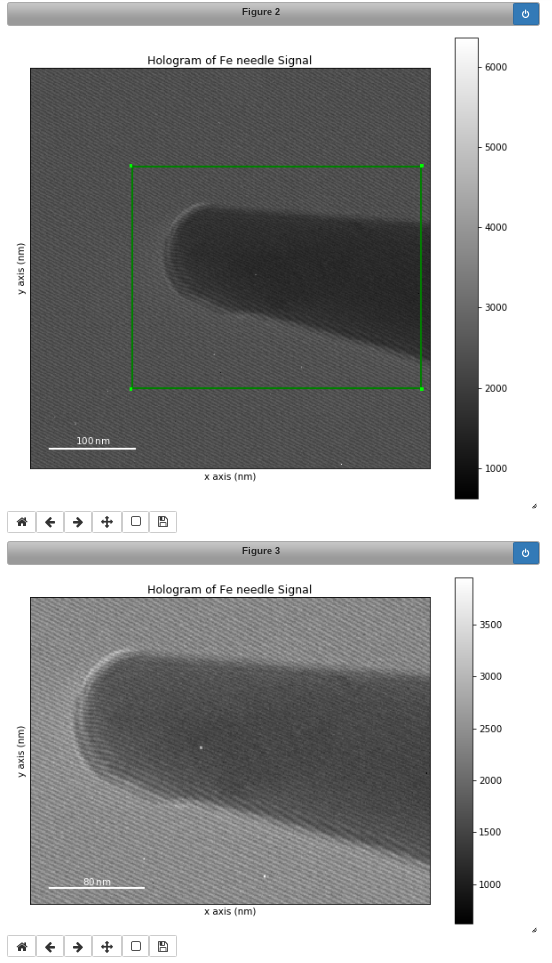# Signal2D Tools¶

The methods described in this section are only available for two-dimensional signals in the `Signal2D`. class.

## Signal registration and alignment¶

The `align2D()` and `estimate_shift2D()` methods provide advanced image alignment functionality.

```# Estimate shifts, then align the images
>>> shifts = s.estimate_shift2D()
>>> s.align2D(shifts=shifts)

# Estimate and align in a single step
>>> s.align2D()
```

Warning

`s.align2D()` will modify the data in-place. If you don’t want to modify your original data, first take a copy before aligning.

Sub-pixel accuracy can be achieved in two ways:

• scikit-image’s upsampled matrix-multiplication DFT method [Guizar2008], by setting the `sub_pixel_factor` keyword argument

• for multi-dimensional datasets only, using the statistical method [Schaffer2004], by setting the `reference` keyword argument to `"stat"`

```# skimage upsampling method
>>> shifts = s.estimate_shift2D(sub_pixel_factor=20)

# stat method
>>> shifts = s.estimate_shift2D(reference="stat")

# combined upsampling and statistical method
>>> shifts = s.estimate_shift2D(reference="stat", sub_pixel_factor=20)
```

If you have a large stack of images, you can perform the image alignment step in parallel by passing `parallel=True`. You can control the number of threads used with the `max_workers` argument. See the map documentation for more information.

```# Estimate shifts
>>> shifts = s.estimate_shift2D()

# Align images in parallel using 4 threads
>>> s.align2D(shifts=shifts, parallel=True, max_workers=4)
```

## Cropping an image¶

The `crop_image()` method crops the image in-place e.g.:

```>>> im = hs.datasets.example_signals.object_hologram()
>>> imc = im.crop(left=120, top=300, bottom=560) # im is cropped in-place
```

Cropping in HyperSpy is performed using the Signal indexing syntax. For example, to crop an image:

```>>> im = hs.datasets.example_signals.object_hologram()
>>> # im is not cropped, imc is a "cropped view" of im
>>> imc = im.isig[120.:, 300.:560.]
```

It is possible to crop interactively using Region Of Interest (ROI). For example:

```>>> im = hs.datasets.example_signals.object_hologram()
>>> roi = hs.roi.RectangularROI(left=120, right=460., top=300, bottom=560)
>>> im.plot()
>>> imc = roi.interactive(im)
>>> imc.plot()
```Interactive image cropping using a ROI.

A linear ramp can be added to the signal via the `add_ramp()` method. The parameters ramp_x and ramp_y dictate the slope of the ramp in x- and y direction, while the offset is determined by the offset parameter. The fulcrum of the linear ramp is at the origin and the slopes are given in units of the axis with the according scale taken into account. Both are available via the `AxesManager` of the signal.

## Peak finding¶

New in version 1.6.

The `find_peaks()` method provides access to a number of algorithms for peak finding in two dimensional signals. The methods available are:

### Maximum based peak finder¶

```>>> s.find_peaks(method='local_max')
>>> s.find_peaks(method='max')
>>> s.find_peaks(method='minmax')
```

These methods search for peaks using maximum (and minimum) values in the image. There all have a `distance` parameter to set the minimum distance between the peaks.

• the `'local_max'` method uses the `skimage.feature.peak_local_max()` function (`distance` and `threshold` parameters are mapped to `min_distance` and `threshold_abs`, respectively).

• the `'max'` method uses the `find_peaks_max()` function to search for peaks higher than `alpha * sigma`, where `alpha` is parameters and `sigma` is the standard deviation of the image. It also has a `distance` parameters to set the minimum distance between peaks.

• the `'minmax'` method uses the `find_peaks_minmax()` function to locate the positive peaks in an image by comparing maximum and minimum filtered images. Its `threshold` parameter defines the minimum difference between the maximum and minimum filtered images.

### Zaeferrer peak finder¶

```>>> s.find_peaks(method='zaefferer')
```

This algorithm was developed by Zaefferer [Zaefferer2000]. It is based on a gradient threshold followed by a local maximum search within a square window, which is moved until it is centered on the brightest point, which is taken as a peak if it is within a certain distance of the starting point. It uses the `find_peaks_zaefferer()` function, which can take `grad_threshold`, `window_size` and `distance_cutoff` as parameters. See the `find_peaks_zaefferer()` function documentation for more details.

### Ball statistical peak finder¶

```>>> s.find_peaks(method='stat')
```

Described by White [White2009], this method is based on finding points that have a statistically higher value than the surrounding areas, then iterating between smoothing and binarising until the number of peaks has converged. This method can be slower than the others, but is very robust to a variety of image types. It uses the `find_peaks_stat()` function, which can take `alpha`, `window_radius` and `convergence_ratio` as parameters. See the `find_peaks_stat()` function documentation for more details.

### Matrix based peak finding¶

```>>> s.find_peaks(method='laplacian_of_gaussians')
>>> s.find_peaks(method='difference_of_gaussians')
```

These methods are essentially wrappers around the Laplacian of Gaussian (`skimage.feature.blob_log()`) or the difference of Gaussian (`skimage.feature.blob_dog()`) methods, based on stacking the Laplacian/difference of images convolved with Gaussian kernels of various standard deviations. For more information, see the example in the scikit-image documentation.

### Template matching¶

```>>> x, y = np.meshgrid(np.arange(-2, 2.5, 0.5), np.arange(-2, 2.5, 0.5))
>>> template = hs.model.components2D.Gaussian2D().function(x, y)
>>> s.find_peaks(method='template_matching', template=template)
```

This method locates peaks in the cross correlation between the image and a template using the `find_peaks_xc()` function. See the `find_peaks_xc()` function documentation for more details.

## Interactive parametrization¶

Many of the peak finding algorithms implemented here have a number of tunable parameters that significantly affect their accuracy and speed. The GUIs can be used to set to select the method and set the parameters interactively:

```>>> s.find_peaks(interactive=True)
```

Several widgets are available:

• The method selector is used to compare different methods. The last-set parameters are maintained.

• The parameter adjusters will update the parameters of the method and re-plot the new peaks.

Note

Some methods take significantly longer than others, particularly where there are a large number of peaks to be found. The plotting window may be inactive during this time.